Numerical Relativistic Hydrodynamics

Hydrodynamics allows for an effective description of macroscopic matter providing the matter is in local thermal equilibrium.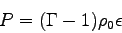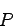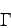Therefore the state of a patch of fluid is fully described by a set of position dependent state variables (i.e. fields). The evolution of these fields is governed by the relativistic Euler's equations. In addition to the equations of motion one must supply the eqaution of state. The equation of state for the ideal fluid has the form where is the isotropic pressure is the rest mass density and is the specific internal energy. is the adiabatic index (). Euler's equations constitute a set of hyperbolic partial differential equations. By specific choice of variables these equations can be written in conservative form (possibly with sources). It is well known that solutions of the Euler's equations generically develop discontinuities even from initially smooth data. One can either smooth the discontinuities by introducing an artificial viscosity or use a different method for solving the hydrodynamic equations - the so called high resolution shock capturing methods (HRSC). In recent years the latter approach became increasingly more popular. HRSC methods are based on a different type of discretization - the so-called finite volume discretization. Here instead of approximating the value of a field variable at a grid point one evolves the volume average of  the field over a computational cell. One then calculates the fluxes of the conservatives fluid variables over all of the cell boundaries and  then adjusts the cell averages accordingly (if sources are present their contribution is also included) Since the Einstein equations are most often solved with finite difference approximation it is not straightforward to incorporate matter into the calculations. I am currently involved in a collaborative effort with Frans Pretorius to include the HRSC hydro solver into his parallel adaptive mesh refinment infrastructure (PAMR/AMRD). More about this can be found here.
In some cases one can assume that the fluid is not coupled to gravity (a test fluid) and only moves in a prescribed geometry. This geometry is typically a static or stationary solution of Einstein's eqautions such as Minkowski, Schwarzschild or Kerr spacetime. This approach has been successfully used to simulate astrophysical jets, wind accretion onto black holes and accretion disc around black holes. In order to familiarize myself with the HRSC methods in the framework of GR I have developed a code which can evolve relativistic ideal fluid in a background geometry in 1D,2D (spherical symmetry, axisymmetry) and 3D. The code can run in a parallel environment (using PAMR) and performs well on a Linux Beowulf cluster.

Astrophysical Jets

Jets are ubiquitous in extragalactic radio sources associated with active galactic nuclei. Although to explain their origin GR magnetohydrodynamics is needed, to study the morphology of the jet a SR hydrodynamics is sufficient. The following is a simulation of a 2D planar jet (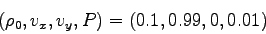) moving through an extragalactic medium (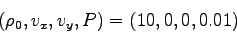). The first image/movie below shows the log of the fluid density of a the jet. The second image/movie shows a 3D view of the domain. In order to visualize the fluid flow one can trace test particles trajectories. I started with random uniform particle distribution and regularly added particles into the jet source. The third image/movie shows the motion of the tracers. Since the jet is symmetric it is sufficient only to use half of the domain for calculations and to use reflection boundary conditions at the symmetry axis. The computational domain is (-20,55)x(0,21) and the resolution is 3000x840 cells.
Note the presence of an external  bow shock and the vortices due to the Kelvin-Helmholtz instability.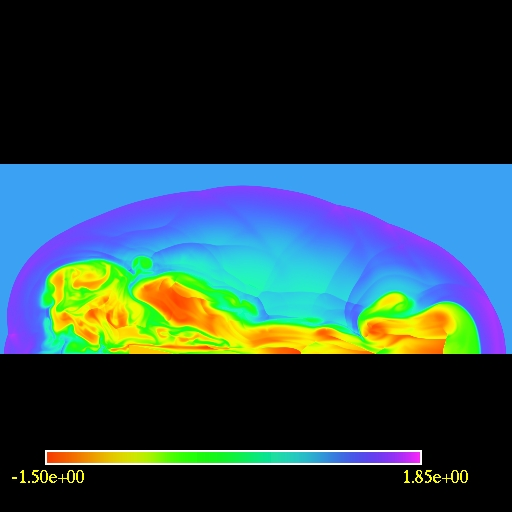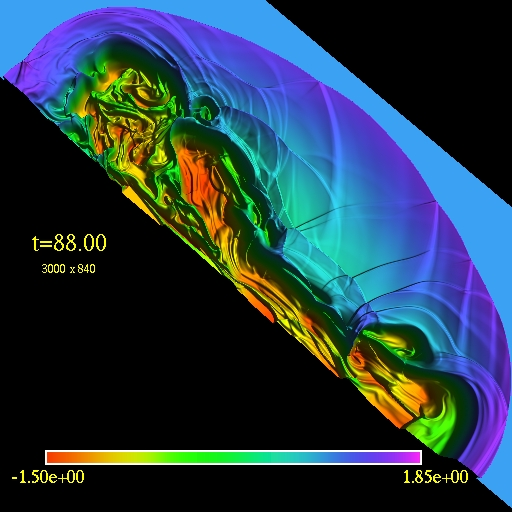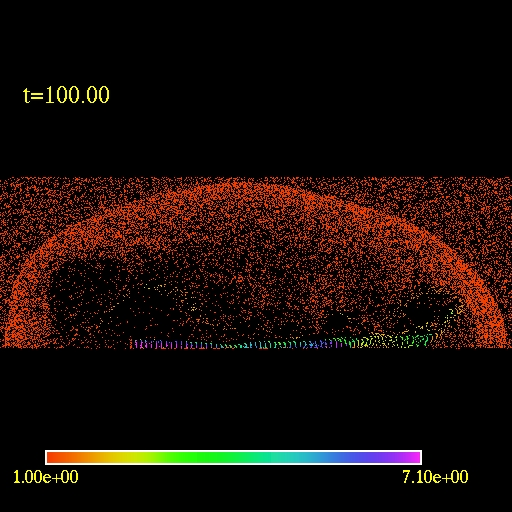2D view of the computational domain. Logarithm of the fluid density  is plotted. (click to play mpeg movie) 3D view of the computational domain. Logarithm of the fluid density  is plotted. (click to play mpeg movie) Visualization of the fluid flow  by trace particles. (click to play mpeg movie)

Wind Accretion onto Black Hole

These are the results of simulations of a relativistic wind accretion onto a Schwarzschild BH. The models are taken from the paper by Font et. al.
A NUMERICAL STUDY OF RELATIVISTIC BONDI-HOYLE ACCRETION ONTO A MOVING BLACK HOLE:AXISYMMETRIC COMPUTATIONS IN A SCHWARZSCHILD BACKGROUND,494,297-316 (1998)

Here is the table from the paper describing the models (I think that rmin(ra) for model MC2 should be 0.57 - 0.44 would be below the Schwarzschild radius)

 Model cs∞ G Μ∞ v∞ ra(M) rmin(ra) rmax(ra) tf(M) MA1... 0.1 1.1 1.5 0.15 30.8 0.125 10.0 4000 MA2... 0.1 1.1 5.0 0.5 3.8 0.57 10.0 750 MB1... 0.1 4/3 1.5 0.15 30.8 0.125 10.0 4000 MB2... 0.1 4/3 5.0 0.5 3.8 0.57 10.0 750 MC1... 0.1 5/3 1.5 0.15 30.8 0.125 10.0 2000 MC2... 0.1 5/3 5.0 0.5 3.8 0.44 10.0 750 UA1... 0.31 1.1 1.5 0.47 3.2 0.69 9.38 200 UA2... 0.31 1.1 3.0 0.93 1.04 2.12 28.85 110 UB0... 0.57 4/3 0.6 0.34 2.2 1.0 13.64 200 UB1... 0.57 4/3 1.5 0.86 0.92 2.39 32.61 200 UC0... 0.81 5/3 0.6 0.49 1.1 2.0 27.27 200

 cs∞ asymptotic sound speed (i.e., at the outer boundary) G adiabatic  exponent Μ∞ asymptotic Mach number at infinity v∞ asymptotic flow velocity ra(M) accretion radius rmin(ra) inner boundary in units of accretion radius rmax(ra) outer boundary in units of accretion radius tf(M) final time at which the simulation was stopped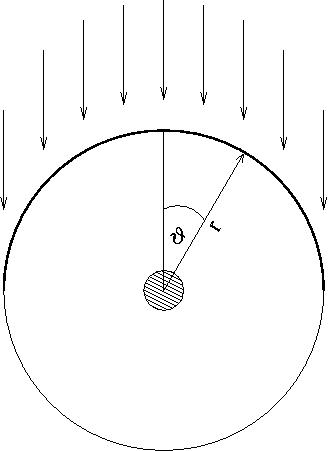Wind accreation schematics. The outer circle is the outer boundary. The small circle in the middle represents the inner boundary with the black hole.

First is the simulation of the model MC2 performed on a 200x100 grid (r,theta) using Eddington-Finkelstein ingoing coordinates. The grid is logarithmic in r-direction and uniform in theta. The inner radius is located at r=1.75M and the outer radius is at r=100M. In this simulation (unlike in the paper) I put vacuum in the whole domain except at the ghost zones in the upper hemisphere at initial time. The vacuum was a true vacuum, i.e., all the variables were set to zero. It is a bit tricky to evolve such a setup but the method I implemented seemed to work fine. Since this is log10 plot I added 1.e-3 to the density so vacuum would be represented by the value -3.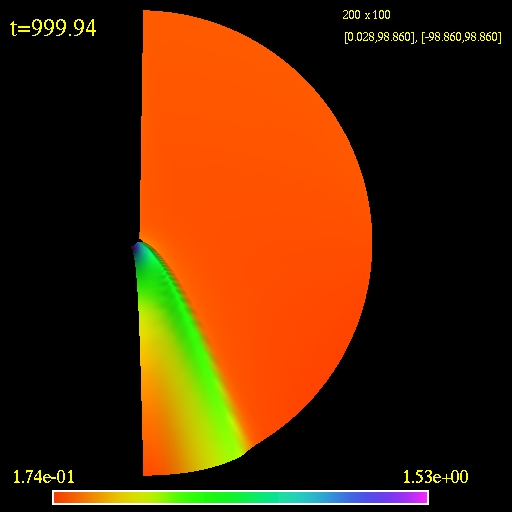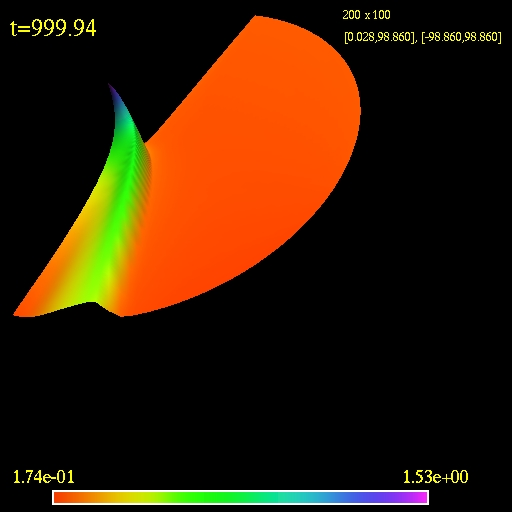2D view of the computational domain. Logarithm of the fluid density  is plotted. (click to play mpeg movie) 3D view of the computational domain. Logarithm of the fluid density  is plotted. (click to play mpeg movie)

The next simulation is for the model MB1. This particular one is done in Schwarzschild coordinates but the results do not change when using Eddington-Finkelstein ingoing coordinates. The simulation shows that if a stationary state is reached it would be qualitatively different than the one for the model MC2 which is unexpected. I performed many simulations in both types of coordinate systems with different resolutions, domain sizes and  flux  approximations. All  result  in the same behaviour, i.e., the detachment of the initially formed shock and its dispersion. My computed accretion rates for all tested models agree to those published until the  tf(M) for each simulation.  My evolution of the model MC1 shows similar behaviour - a hint of it might be even present in the paper - the simulation of C1 shows a detached shock but the simulation was stopped at t=2000M.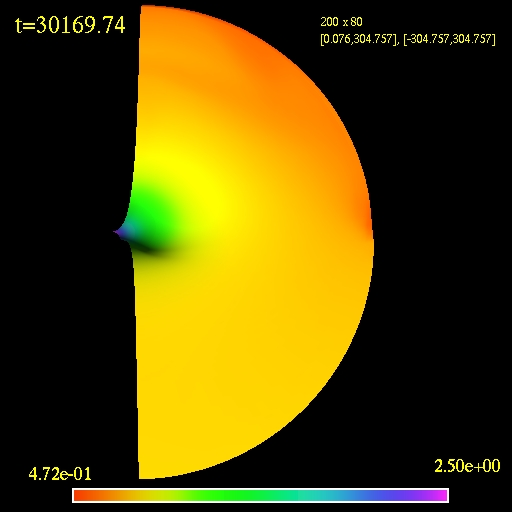2D view of the computational domain. Logarithm of the fluid density  is plotted. (click to play mpeg movie) 3D view of the computational domain. Logarithm of the fluid density  is plotted. (click to play mpeg movie)

Accretion Discs

The most spectacular accretion discs in nature are found in active galactic nuclei and most probably at the center of quasars. It is thought that a super massive black hole is located at the center with matter orbiting around it and spiraling inwards. Below are two simulations of accretion discs around Schwarzschild black hole. The first image/movie shows an evolution of an stable accretion disc. All of the matter is inside its Roche lobe so no matter transfer should occur (a "numerical leak" can be seen at the end of the simulation).
The second image/movie shows an evolution of a disc overflowing its Roche lobe. There is a steady matter flow into the black hole. The model s are taken from arxiv:astro-ph/0203403.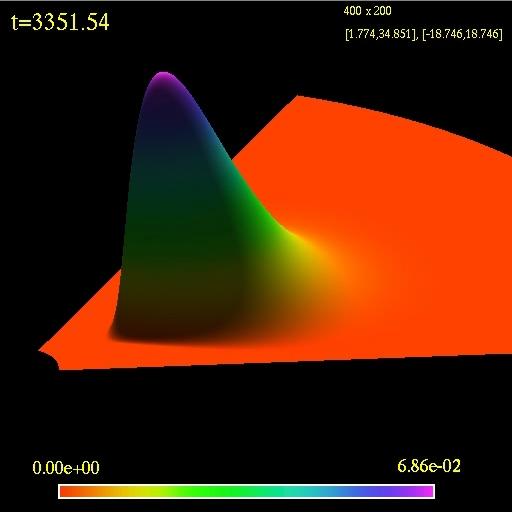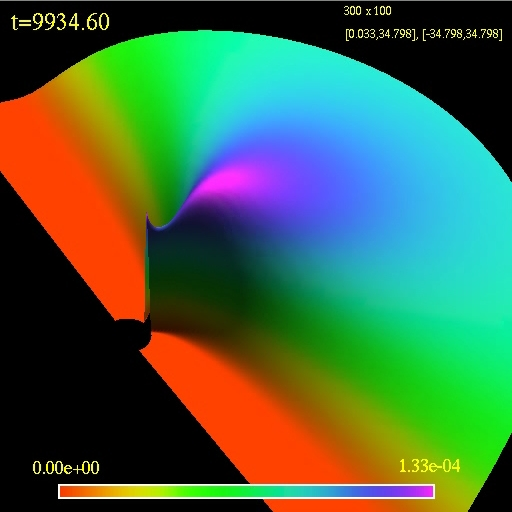3D view of the computational domain. Logarithm of the fluid density  is plotted. (click to play mpeg movie) 3D view of the computational domain. Logarithm of the fluid density  is plotted. (click to play mpeg movie)